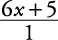## Examples of Rational Expressions

Rational expression is a fancy way of saying fraction. Of course, a fraction also may be perceived as being a division example, wherein the numerator is being divided by the denominator. Although the basic rules of arithmetic of fractions apply to the rational expressions treated within this chapter, having polynomials in the numerators and/or denominators does, at times, require some fancy footwork. This is even more true when a fraction is in the numerator and another one is in the denominator (a complex fraction).

The quotient of two polynomials is a rational expression. The denominator of a rational expression can never have a zero value. The following are examples of rational expressions:The last example, 6 x + 5, could be expressed asTherefore, it satisfies the definition of a rational expression.